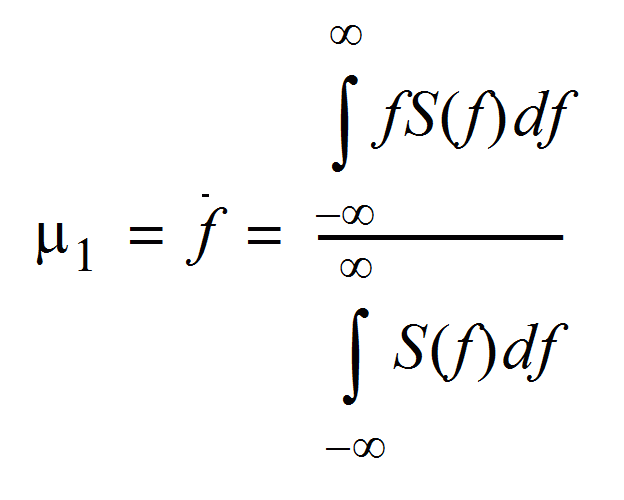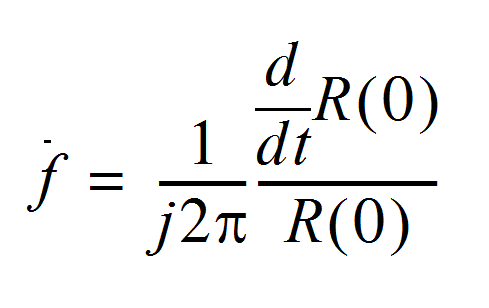# Measuring transient flows

## Theoretical basis of the Doppler frequency estimation

The amplitudes of the echoes reflected by the particles within the flowing fluid are somewhat random in nature, corresponding to the random distribution of the particles in the fluid medium. Thus, the Doppler signals may be treated as random processes, and characterized by different moments.

In order to be able to determine the probability of occurrence of this process, one must have access to a great number of actual occurrences of the process. In practice, it is difficult to obtain measurements of the exact same process under the exact same conditions at several different times. Therefore, a temporal average is preferable to an ensemble average.

The temporal average and the ensemble average will not be the same unless the process is stationary and the analysis time is very long (tending to infinity). Considering the Doppler process as stationary, the average frequency may be expressed as the normalized first moment, where S(f) is the spectral density or probability density of the Doppler signal.The Doppler frequency computation algorithm is based on the fact that the inverse Fourier transform of the probability density of a stationary process is equal to the auto-correlation function.

The mean Doppler frequency may be expressed in terms of the time derivatives of the auto-correlation function at the origin.

Transient flows are characterized by variations in velocities versus time. The non-stationary propriety of transient flows implies that a bias will be introduced in the time derivatives of the auto-correlation function. The computation of the auto-correlation could only be realized during a finite time interval and only samples of the analytical signal are available (spaced in time by PRF).

## How to measure transient flows

In order to reduce the bias induced by the non-stationary propriety of transient flows, the number of emissions used to compute the mean Doppler frequency must be reduced. Unfortunately reducing the number of emissions will decrease the quality of the estimation of the auto-correlation function. A comprise must be therefore realized between the number of emissions used and the quality of the estimated mean Doppler frequency.

A low number of emissions pro profile increases the variance in the measured mean Doppler frequency. This variance can be reduced by using a moving average filter.